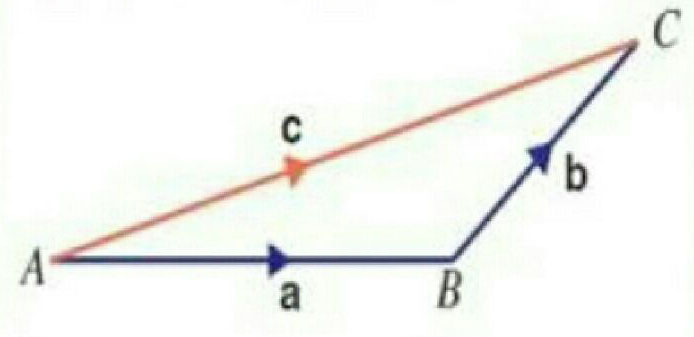Q

# Help me answer: If the magnitude of sum of two vectors is equal to the magnitude of difference of the two vectors, the angle between these vectors is:

If the magnitude of sum of two vectors is equal to the magnitude of difference of the two vectors, the angle between these vectors is:

• Option 1)

0o

• Option 2)

90o

• Option 3)

45o

• Option 4)

180o

1930 Views

As we learned in

Triangle law of vector Addition -

If two vector are represented by both magnitude and direction by two sides of triangle taken in same order then their resultant is represented by 3rd side of triangle.

- whereinRepresents triangle law of vector Addition

Option 1)

0o

This option is incorrect.

Option 2)

90o

This option is correct.

Option 3)

45o

This option is incorrect.

Option 4)

180o

This option is incorrect.

Exams
Articles
Questions